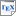[CHK13] T. Chen, T. Han, M. Kwiatkowska. On the Complexity of Model Checking Interval-valued Discrete Time Markov Chains. Information Processing Letters, 113(7), pages 210-216, Elsevier. 2013. [pdf] [bib] Downloads:pdf (213 KB)bib Links: [Google] [Google Scholar] [CiteSeer] Abstract. We investigate the complexity of model checking (finite) interval-valued discrete time Markov chains, that is, discrete time Markov chains where each transition is associated with an interval in which the actual transition probability must lie. Two semantics are considered, the uncertain Markov chain (UMC) semantics and the interval Markov decision process (IMDP) semantics. We show that, for reachability, these two semantics coincide and the problem is P-complete. This entails that PCTL model checking problem under the IMDP semantics is also P-complete. We also show that model checking PCTL under the UMC semantics is Square-Root-Sum hard, meaning that one can reduce the Square-Root-Sum problem to it.